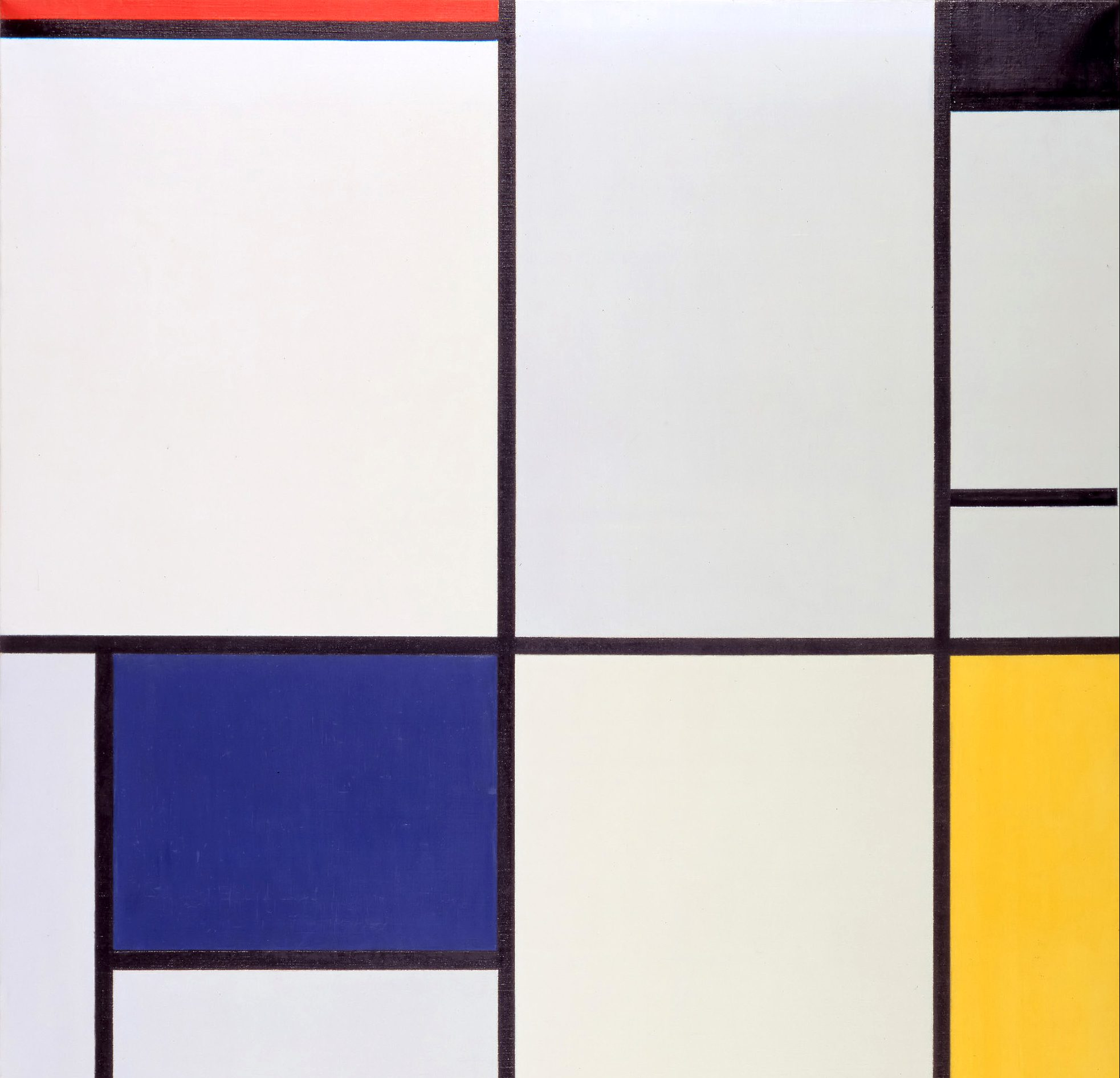# The popularitygap

Combinatorics

A finite nonempty subset A of a cyclic group, with small enough |A–A|, contains a nonzero element with at least (2+o(1))|A|²/|A–A| representations as a difference of two elements.

## The popularity gap

In press Journal of Algebraic Combinatorics

V. F. Lev, I. Shkredov

Suppose that A is a finite, nonempty subset A of a cyclic group of either infinite or prime order. We show that if the difference set A − A is “not too large”, then there is a non zero group element with at least as many as (2 + o(1))|A|^2/|A − A| representations as a difference of two elements of A; that is, the second largest number of representatives is, essentially, twice the average. Here the coefficient 2 is best possible. We also prove continuous and multidimensional versions of this result, and obtain similar results for sufficiently dense subsets of an arbitrary abelian group.

In press Journal of Algebraic Combinatorics

V. F. Lev, I. Shkredov